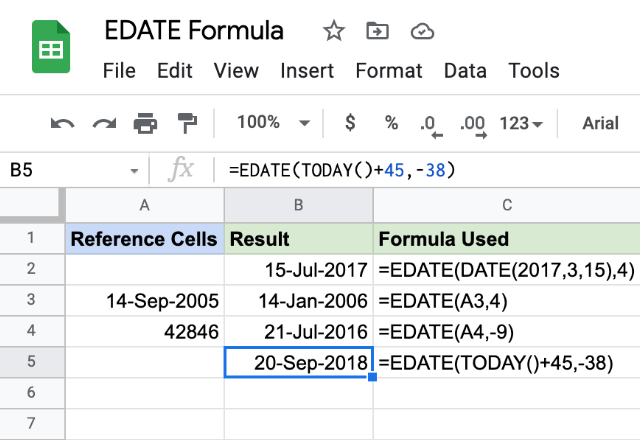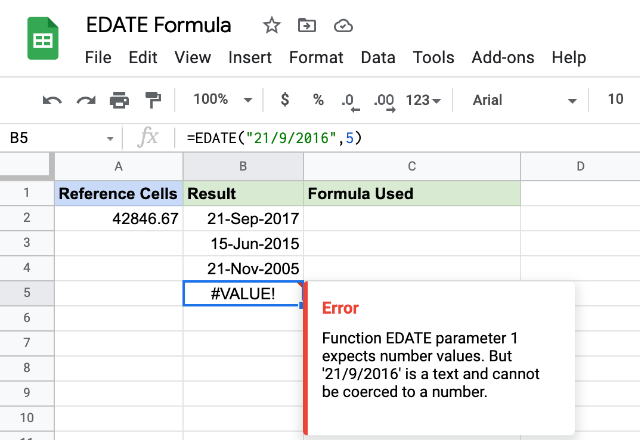# How to use the EDATE formula in Google SheetsIn Google Sheets application, if we need to know the date a specified number of months before or after a given date, the EDATE formula can help us do so.

### Syntax

EDATE(start_date, months)

• start_date – is the date that the EDATE formula calculates the prior or later date separated by the specified number of months.
• months – is the number of months that the formula should move either in forward or backward in time, corresponding to a positive value or a negative value respectively.

### Usage: EDATE Formula

Let us understand it’s working by making use of a few examples. Please consider the snapshot below. Please note that we have preset the Result column format to “dd-mmm-yyyy”, for the purposes of avoiding ambiguity (given that the date notations varies across countries).In the first example case, the start_date parameter takes in the output that the DATE() function returned. And we have set the months parameter to ‘4’. Therefore, the formula calculates the result date that is 4 months ahead of 15-Mar-2017.

The second example is pretty similar, except for the start_date parameter, we provided a reference to the cell that has a date.

Unlike the previous two cases, the start_date for the third case is an absolute number. And also, we have keyed in a negative value for the month’s parameter. The EDATE formula calculates the resulting date by shifting the time from the date value 42846 (equivalent to 21-Apr-2017) back by nine months.

Let us take a look at the fourth case. At the time of writing this post, the date was 21-Apr-2017 (which, the TODAY() function evaluated to in the second example). Factoring a shift of 45 days we added to the TODAY() function, along with a value of -38 for months parameter, we get the last date of the month as 05-Apr-2014.

##### Cases of interest

Let us experiment a little further and input text based date values for the start_dateparameter.In the first example, the formula truncates the decimal values and uses only the integer values and accordingly evaluates the resulting date. Of course, it cannot accept negative numbers, as there is no date interpretation for such values.

From the next three examples, we will that the EDATE formula accepts input date strings in the “dd-mmm-yyyy” and “mm-dd-yyyy” formats. But it will throw up an error with “dd-mm-yyyy” date format strings.